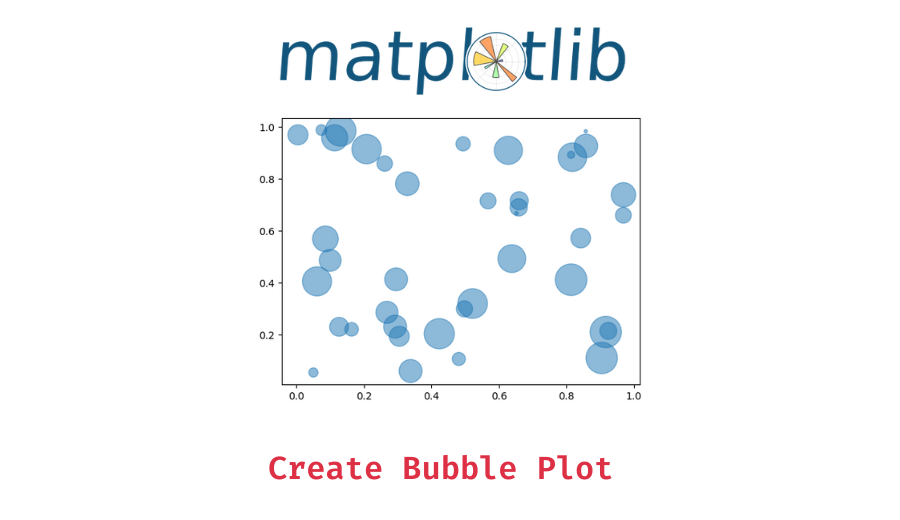# How To Make a Bubble Plot in Python with Matplotlib?

In this tutorial, we’ll try to understand how to make a bubble plot in python using the matplotlib library.

A bubble plot is a scatterplot, but with the size of the data points on the scatter plot represented by another variable. Basically, if the size variable is larger you get a bigger circle filled with a color i.e. bigger bubble, and similarly, a smaller bubble for a smaller numerical value.

## Using the `matplotlib.pyplot.scatter()` method

You can use the matplotlib pyplot module’s `pyplot.scatter()` method to create a bubble plot in Python. The idea is to create a scatter plot with the size of the data points dependent on another variable which you can provide using the `s` parameter.

You can provide an array or list of values to use as the marker size for the different data points.

Basic Syntax:

`matplotlib.pyplot.scatter(x, y, s=None, c=None, **kwargs)`

Parameters:

• x, y-float or array-like, shape (n, ): The data positions.
• s-float or array-like, shape (n, ), optional: The marker size in points**2 (typographic points are 1/72 in.).
• c-array-like or list of colors or color, optional: The marker colors.

For more parameters, refer this.

Now let us understand the above method with some worked out examples

📚 Data Science Programs By Skill Level

Introductory

Intermediate ⭐⭐⭐

🔎 Find Data Science Programs 👨‍💻 111,889 already enrolled

Disclaimer: Data Science Parichay is reader supported. When you purchase a course through a link on this site, we may earn a small commission at no additional cost to you. Earned commissions help support this website and its team of writers.

### Example 1 – Simple bubble plot

```import matplotlib.pyplot as plt
import numpy as np

x = np.random.rand(40)
y = np.random.rand(40)
z = np.random.rand(40)

# use the scatter function
plt.scatter(x, y, s=z*1000, alpha=0.5)

# show the graph
plt.show()```

Output:

In the above example, we –

1. Import the required modules.
2. Generate random x,y, and z values where x&y values work as the coordinates for the scattering points and the z values represent the size of the markers with which the scatter points are plotted.
3. Plot the points with the respective marker size and display the plot.

### Example 2 – Bubble plot with colors

```import matplotlib.pyplot as plt
import numpy as np

x = np.random.rand(40)
y = np.random.rand(40)
z = np.random.rand(40)
colors = np.random.rand(40)

# use the scatter function
plt.scatter(x, y, s=z*1000,c = colors, alpha=0.5)

# show the graph
plt.show()```

Output:

In the above example, we –

1. Import the required modules.
2. Generate random x,y, and z values where x&y values work as the coordinates for the scattering points and the z values represent the size of the markers with which the scatter points are plotted.
3. Generate random colors for each point.
4. Plot the points with the respective marker size and color and display the plot.

### Example 3 – Bubble plot with a different shape

You can also customize the shape of the bubble. Since we’re essentially plotting a scatter plot, you can customize the shape of the points (or bubbles) by changing the marker shape itself. For example, let’s plot a bubble plot with diamond-shaped bubbles.

```import matplotlib.pyplot as plt
import numpy as np

x = np.random.rand(10)
y = np.random.rand(10)
z = np.random.rand(10)

# use the scatter function
plt.scatter(x, y, s=z*1000,marker='D',alpha=0.5)

# show the graph
plt.show()```

Output:

In the above example, we –

1. Import the required modules.
2. Generate random x,y, and z values where x&y values work as the coordinates for the scattering points and the z values represent the size of the markers with which the scatter points are plotted.
3. Plot the points with the respective marker size and specify the marker shape using `marker='D'` and display it.

For more details about the different markers used, refer this.

### Example 4 – Bubble plot with more customizations

You can similarly further customize your bubble plot by using additional parameters in the `pyplot.scatter()` function, for example, let’s change the line width of the markers and make its content slightly transparent.

```import matplotlib.pyplot as plt
import numpy as np

x = np.random.rand(10)
y = np.random.rand(10)
z = np.random.rand(10)

# use the scatter function
plt.scatter(x, y, s=z*1000,marker='D',alpha=0.5,linewidth=5)

# show the graph
plt.show()```

Output:

You might also be interested in –

•